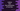# How to find the intersection of two list elements in python## Introduction :

In this python programming tutorial, we will learn how to find the intersection of two lists. The program will create two lists by taking the inputs from the user first. It will then find out the intersection of the lists and print out the result.

### Algorithm to use :

1. Create two empty list variables.
2. Ask the user to enter the size of both lists. Store the sizes in two different variables.
3. Using one for loop, take the user inputs for both of these lists.
4. Find the intersection of the list and store it in a different variable.
5. Print the intersection to the user.

### set conversion :

To solve this problem, we will first convert the list into a set using set() function. Python list doesn’t have any inbuilt method to do the intersection, so we are converting the list to a set. After the list is converted to a set, we can easily calculate the intersection by using the & operator. Then, we can convert the final set to a list by using list() method.

## Python program :

``````#1
list1 = []
list2 = []

#2
size1 = int(input("Enter the size of the first list : "))
size2 = int(input("Enter the size of the second list : "))

#3
print("For the first list : ")
for i in range(0, size1):
e = int(input("Enter element for position {} : ".format(i)))
list1.append(e)

#4
print("For the second list : ")
for i in range(0, size2):
e = int(input("Enter element for position {} : ".format(i)))
list2.append(e)

#5
list_intersection = list(set(list1) & set(list2))

#6
print("Intersection of {} and {} is : {}".format(
list1, list2, list_intersection))``````

### Explanation :

The commented numbers in the above program denote the step numbers below :

1. Create two empty list list1 and list2.
2. Ask the user to enter the size of the first list. Read it and store it in variable size1. Similarly, read the size of the second list and store it in size2.
3. Take the user inputs for the first list using a for loop. Ask the user to enter the element for each position in the for loop. Read it and store it in variable ‘e’. Append this value to list1.
4. Similar to the above step, read and append all values to the second list list2.
5. Find out the intersection of list1 and list2. Use set() method to convert each list to set. Then use ’&’ operator to calculate the intersection of both set. Finally, use list() method to convert the intersection set to a list. Store the intersection list in variable listintersection_.
6. Print out the result to the user.

### Sample output :

``````Enter the size of the first list : 3
Enter the size of the second list : 4
For the first list :
Enter element for position 0 : 1
Enter element for position 1 : 2
Enter element for position 2 : 3
For the second list :
Enter element for position 0 : 2
Enter element for position 1 : 3
Enter element for position 2 : 4
Enter element for position 3 : 5
Intersection of [1, 2, 3] and [2, 3, 4, 5] is : [2, 3]

Enter the size of the first list : 4
Enter the size of the second list : 4
For the first list :
Enter element for position 0 : 1
Enter element for position 1 : 23
Enter element for position 2 : 45
Enter element for position 3 : 67
For the second list :
Enter element for position 0 : 23
Enter element for position 1 : 46
Enter element for position 2 : 45
Enter element for position 3 : 55
Intersection of [1, 23, 45, 67] and [23, 46, 45, 55] is : [45, 23]``````

### Conclusion :

For doing a set operation like intersection, union of list elements, it is a quick process to convert them to ‘set’ first. We can also use loops to iterate over the elements of both lists and find out the intersection, but that will be a time-consuming process and may not be a wise decision to write down one new method only for the intersection.